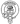# How to compare two arrays in JavaScript

August 14, 2020

If you want to compare two arrays in JavaScript it’s not a simple as just chucking a comparison operator in between them. Here’s a simple example:

``````const array1 = ['a', 'b', 'c', 'd']
const array2 = ['a', 'b', 'c', 'd']

console.log(array1 == array2)

// false``````

Despite both our arrays containing the same elements in the same positions the comparison still returns as false. To compare an array we instead need to loop through and compare every value.

``````const arrayIsEqual = (a, b) => {
// Ensure length is the same, a great timesaving optimization
if (a.length != b.length) return false

for (let i = 0, len = a.length; i < len; i++) {
// Is there nested arrays?
if (a[i] instanceof Array && b[i] instanceof Array) {
// Leverage recursion to check these nested arrays
if (!a[i].equals(b[i])) return false
} else if (a[i] != b[i]) {
return false
}
}
return true
}``````

Now let’s see it in action:

``````const array1 = ['a', 'b', 'c', 'd']
const array2 = ['a', 'b', 'c', 'd']
const array3 = ['b', 'd', 'c', 'd']

console.log(arrayIsEqual(array1, array2))

// true

console.log(arrayIsEqual(array2, array3))

// false``````

It’s also possible to override `Array.prototype.equals` with this function for convenience however I’m not a big fan of doing so as especially in larger code bases with multiple team members this can cause unexpected behavior.

If you’re already using the utility library lodash or underscore.js in your project you can compare arrays by using their built in `isEqual` function.

``````const array1 = ['a', 'b', 'c', 'd']
const array2 = ['a', 'b', 'c', 'd']
const array3 = ['b', 'd', 'c', 'd']

console.log(_.isEqual(array1, array2))

// true

console.log(_.isEqual(array2, array3))

// false``````Written by Fraser Hamilton a full stack developer based out of Edinburgh, Scotland.